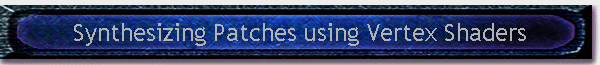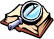#Written by Robert Dunlop Microsoft DirectX MVP< Previous: Page 2 Page 3 Next: Page 4 >

## Computing Vertex Position

To render the vertices in a patch to a specified location using a vertex shader, four basic steps will be involved in the calculation of the vertex position:

1. Calculate height (Y) based on input vertex X and Z, and compose a vector x,y,z.
2. Scale the vector to the specified dimensions of the patch.
3. Translate the vector to the specified origin of the patch in world space.
4. Transform the vector according to current view and projection matrices, and output to screen.

The code for this portion of the shader looks like this:

 Partial shader listing, showing computation of vertex position //c0-c3 world/view/proj matrix //c4 quad size //c5 quad origin //c6-c9 position spline matrix // get coordinates x,x,z,1 -> r0 mov r0,v0.yyxx mov r0.z,v1.y // get height m4x4 r3,v0,c dp4 r0.y,r3,v1 // scale vertex mul r0,r0,c // translate vertex add r0,r0,c // transform vertex to screen m4x4 oPos,r0,c Listing 7

## Computing Vertex Color

Computation of the vertex color is potentially quite complex, and there are many different ways that it could be applied.  Since we are using a shared vertex buffer and deriving the vertex location on the fly, we won't have pre-defined normals to perform lighting with in the vertex format.

The method that is used in the demo is fairly simple.  The application pre-calculates amount of light at each control point, based on a single directional light source plus an ambient offset.  These scalar values are then used to generate pre-calculated matrices in exactly the same way the positional matrices were created, allowing us to compute an interpolated light value at any point in each quad.

The vertex shader generates a material color based upon the height of each vertex, ranging from green at lowest altitude to a reddish-brown at the highest altitude.  To facilitate this, the position splines are all scaled to the range of 0.0 to 1.0, so that the height value derived from the quad can directly be used as an interpolation weight for the vertex color.  The height value is scaled to (1-y)2 to provide a non-linear interpolation between the two colors.

The diffuse color of each vertex is created by multiplying the computed color by the interpolated light intensity.

 Partial shader listing, showing computation of vertex color //c16-c19 lighting spline matrix // define color range def c14,0.7,0.4,0.3,1.0 def c15,-0.6,0.3,-0.2,0.0 ... r0.y derived in Listing 7 ... // calc color sub r7.y,v0.x,r0.y mul r7.y,r7.y,r7.y mul r4,c,r7.y add r4,r4,c // calc light m4x4 r7,v0,c dp4 r7.y,r7,v1 // calculate final diffuse mul oD0,r4,r7.y Listing 8

For information on other ways to perform lighting in this shader, see Potential Improvements.

## The Completed Vertex Shader

Below is the listing of the completed shader, incorporating the elements described above:

 Complete shader listing vs.1.0 // --- shader input ---// //c0-c3 world/view/proj matrix //c4 quad size //c5 quad origin //c6-c9 position spline matrix //c16-c19 lighting spline matrix // --- shader constants --- // // define color range def c14,0.7,0.4,0.3,1.0 def c15,-0.6,0.3,-0.2,0.0 // --- shader code --- // // get coordinates x,x,z,1 -> r0    mov r0,v0.yyxx mov r0.z,v1.y // get height m4x4 r3,v0,c dp4 r0.y,r3,v1 ... continued ... // calc color sub r7.y,v0.x,r0.y mul r7.y,r7.y,r7.y mul r4,c,r7.y add r4,r4,c // calc light m4x4 r7,v0,c dp4 r7.y,r7,v1 // calculate final diffuse mul oD0,r4,r7.y // scale vertex mul r0,r0,c // translate vertex add r0,r0,c // transform vertex to screen m4x4 oPos,r0,c Listing 9
 < Previous: Page 2 Page 3 Next: Page 4 >Table of Contents

Page 1

Page 2 Page 3

Introduction

Streamlining the calculations Computing Vertex Position

Catmull-Rom Splines

Using Vertex Shaders to Render Patches Computing Vertex Color

Surface Representation with Splines

Storage of Common Quad Data

The Completed Vertex Shader
Page 4 Page 5
Setting Up the Landscape The Demo Application
Measuring Height at Arbitrary Locations Potential Improvements
Rendering the LandscapeThis site, created by DirectX MVP Robert Dunlop and aided by the work of other volunteers, provides a free on-line resource for DirectX programmers.

Special thanks to WWW.MVPS.ORG, for providing a permanent home for this site.

#####Visitors Since 1/1/2000:Last updated: 07/26/05.